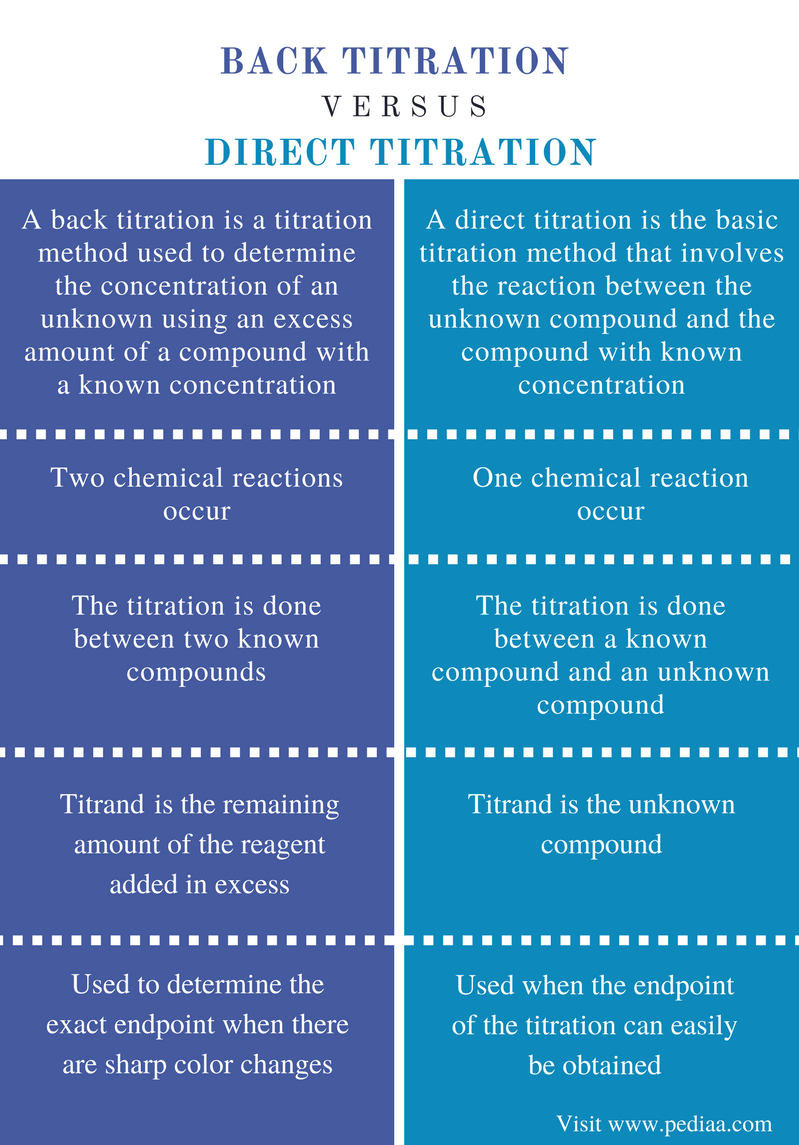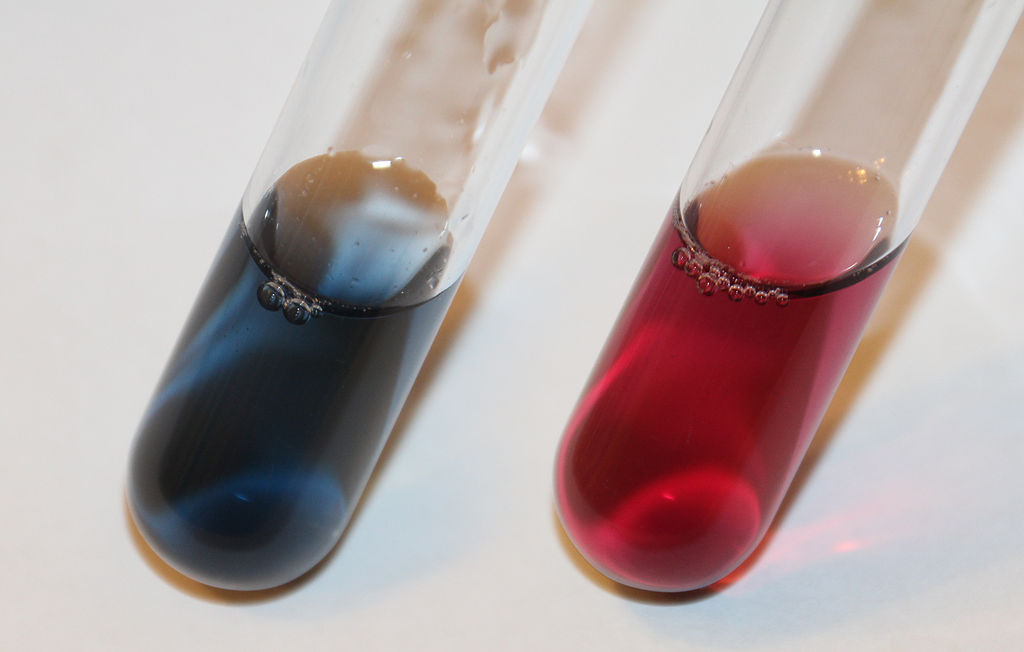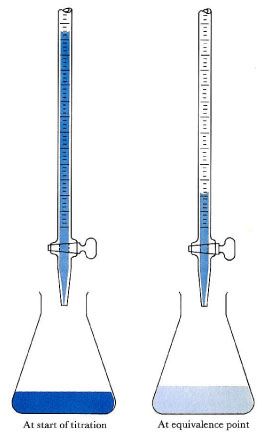# Difference Between Back Titration and Direct Titration

## Main Difference – Back Titration vs Direct Titration

Titrations are chemical techniques used to identify the amount of an unknown compound present in a given mixture. In this technique, we use a solution of a known concentration to find the concentration of an unknown present in our sample. However, we should have an idea about the unknown compound; otherwise, we cannot determine the solution of the known concentration that should be used for this identification. The endpoint of a titration indicates the end of the reaction between the unknown compound and the known compound. Titrations can be done in various ways and there are several types of titrations. Back titrations and direct titrations are two such types. The main difference between back titration and direct titration is that a back titration determines the concentration of the unknown by determining the remaining amount of the compound with a known concentration whereas a direct titration directly measures the concentration of the unknown compound.

### Key Areas Covered

1. What is Back Titration
– Definition, Examples, Applications
2. What is Direct Titration
– Definition, Examples, Applications
3. What is the Difference Between Back Titration and Direct Titration
– Comparison of Key Differences

Key Terms: Back Titration, Direct Titration, EDTA Titrations, End Point, Equivalence Point, Indicator, Titration, Titrand, Titrant## What is Back Titration

A back titration is a titration method used to determine the concentration of an unknown using an excess amount of a compound with a known concentration. There is a chemical reaction between these compounds. Since the amount of the compound with a known concentration added is known already, we can determine the amount of the compound that has reacted with the unknown compound by doing a back titration.

In a titration, there are two components involved: a titrant and a titrand. The titrant is the solution with a known concentration. Titrand is the analyte or the sample. This sample is composed of a compound with an unknown concentration and this compound should react with the titrant solution. A back titration does not involve a direct reaction between the titrant and the titrand. First, we add a compound in excess to the sample solution which can cause a chemical reaction. Then we measure the amount of remaining compound. Therefore, the titrand here is also a known compound.

### Example

Let us consider an example in order to understand this concept. We are given a metal ion solution with an unknown metal ion having an unknown concentration. We can use EDTA back titration method, a common titration method, for the analysis of this solution. Here, an excess amount of EDTA should be first added to the sample solution. The concentration of the EDTA solution should be determined later using a primary standard. The addition of EDTA will cause the formation of metal ion-EDTA complex. Then the remaining amount of EDTA present in the sample is determined using a Mg+2 solution in the presence of EBT indicator. Metal ions always form complexes with EDTA in 1: 1 ratio. Since the previously added amount of EDTA is known, we can calculate the amount of EDTA that reacted with unknown metal.Figure 1: The color change of EBT indicator. The color of free EBT is blue. The metal ion-EBT complex is wine red.

Moreover, a back titration can be used to determine the endpoint of a titration. Sometimes, the end point of titrations cannot easily be obtained due to the sharp color changes that occur at the endpoint. At times like that, we can use a back titration method to obtain the exact endpoint of the titration.

## What is Direct Titration

A direct titration is the basic titration method that involves the reaction between the unknown compound and the compound with known concentration. Here, the addition of excess reagents is not done as in back titrations. The unknown compound is directly reacted with the known compound. Therefore, the end point of the titration indicates the end of the reaction. By using that endpoint, the amount of unknown compound present in the sample solution can be determined.

Most importantly, the end point of the direct titration should be obtained carefully since the endpoint is directly taken for the further calculations. However, the end point of the direct titration does not often give the exact equivalence point of the reaction. This is because the endpoint is given when the indicator used in the titration give its color change. This color change is given a moment after the completion of the reaction. Therefore, it is very important to determine the exact point where the reaction ends.Figure 2: The endpoint of the direct titration can be determined from the color change of the solution.

Acid-base titrations are good examples for direct titrations. Here, an acid is reacted with a base. An indicator is used to determine the end point of the reaction since almost all acids and bases are colorless compounds. With the progression of the reaction, the pH of the solution is changed. At a certain pH, the indicator gives its color change. The point of the color changes is taken as the endpoint of the reaction. Then, we can determine the concentration of the unknown (acid or base) according to the stoichiometric relationship between the acid and the base.

## Difference Between Back Titration and Direct Titration

### Definition

Back Titration: A back titration is a titration method used to determine the concentration of an unknown using an excess amount of a compound with a known concentration.

Direct Titration: A direct titration is the basic titration method that involves the reaction between the unknown compound and the compound with known concentration.

### Reactions

Back Titration: In back titration method, two chemical reactions occur.

Direct Titration: In direct titration method, one chemical reaction occurs.

### Titration

Back Titration: In back titrations, the titration is done between two known compounds.

Direct Titration: In direct titrations, the titration is done between a known compound and an unknown compound.

### Titrand

Back Titration: The titrand of the back titration is the remaining amount of the reagent added in excess.

Direct Titration: The titrand of the direct titration is the unknown compound.

### Applications

Back Titration: Back titrations are used to determine the exact endpoint when there are sharp color changes.

Direct Titration: Direct titrations are used when the endpoint of the titration can easily be obtained.

### Conclusion

Titrations are very useful chemical techniques for identification and quantification of an unknown compound in a sample solution. Back titrations and direct titrations are two types of titration methods. The main difference between back titration and direct titration is that a back titration determines the concentration of the unknown by determining the remaining amount of the compound with a known concentration whereas a direct titration directly measures the concentration of the unknown compound.

##### References:

1. “Titration.” Chemistry LibreTexts, Libretexts, 24 July 2016, Available here. Accessed 18 Sept. 2017.
2. Helmenstine, Ph.D. Anne Marie. “What Is a Back Titration?” ThoughtCo, Accessed 18 Sept. 2017.
3. “What Is “Direct Titration”?” Sciencing, Available here. Accessed 18 Sept. 2017.

Image Courtesy:

1. “Eriochrome Black T indicator color change” By LHcheM – Own work (CC BY-SA 3.0) via Commons Wikimedia
2. “ChemicalPrinciplesFig2-3” By Dickerson, Gray and Haight. The original uploader was Wight at English Wikibooks – (CC BY 3.0) via Commons Wikimedia